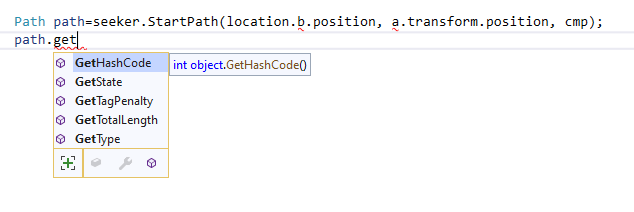# Change speed in Slope and

Hello
1-How can change speed in slopes, Fits the angle?
2-how can get time and cost of one path between two points?(this path can pass through several tags)
3-how can change speed in one tag?
4-how can change penalty of one tag at the runtime?
Thanks

Hi

1. There’s no build in support for this. The normal is not stored as part of the graph. You can use a physics raycast to get the slope angle, though.
``````var path = ABPath.Construct(start, end, null);
path.BlockUntilCalculated();
GetComponent<Seeker>().PostProcess(path);
var totalLength = path.GetTotalLength();
``````
1. You can get the closest node to the agent’s position and adjust its speed based on the tag: `AstarPath.active.GetNearest(myPosition, NNConstraint.Default).node.Tag`.
2. See Graph Updates during Runtime - A* Pathfinding Project

_ I found the slope penalty, the question is: is the delay rate of the slope penalty and the tag penalty added to the TotalLength parameter?

_ Is there a way to create more than 1024 nodes in length and width for the grid graph? (even by change in core, please help me)

The total length is the world space length of the path, not the cost of it. SO tags and other penalties do not affect it.

Yes, this is just an artificial limit. I would not really recommend increasing it, though, because large grid graphs use a lot of memory. You can find the relevant code by opening GridGenerator.cs and searching for “1024”.

1 Like

Thanks so much
now how can the cost of a path be calculated?
For example, part of the path passes the tag penalty and another part of the path passes the slope penalty, what is the total cost of this path?

i found this
GetTraversalCost
Is it possible to get the cost of the whole route by this command and calculating the path vector points.node ?

Dear aron granberg help please …

If you have a path object, you can get the traversal cost using `path.GetTraversalCost`. However there is currently no out of the box method for getting the whole cost for a path (only the world length).

Penalties and tags are summed. So the cost would be the sum.

1 Likepath.GetTraversalCost not exist in this form
but i try to get Nearest of node of each vector pos , and then get Traversal Cost of them and by calculate of vector3.Distance of between them and multiply to cost of them, get cost of path
Is this correct or is it wrong?

Hello anybody

Hi

Hm, sorry, I think the GetTraversalCost is only a public function in the beta version.

The total cost for the path is calculated as:

``````var cost = 0;
for (int i = 0; i < path.path.Count; i++) {
cost += path.GetTraversalCost(path.path[i]);
if (i + 1 < path.path.Count) {
var dist = (path.path[i+1].position - path.path[i].position).costMagnitude;
cost += dist;
}
}
``````
1 Like

Hello

I changed 1024 to 10240 but when i use more than 1024 in the inspector(for example 2048) computer strongly slow down and cpu usage strongly increase in one core and other cores free !

what happened?! what is the problem?!
my computer has many memory(ram) but that is not used and computer make cpu on the one core bounding

if problem is for this is that you make array in one thread, can break array to more than one and make more thread

please guide me for solve it in source

thanks

hello hello
i am waiting hereHi

You can try out the beta version (A* Pathfinding Project) it scans grid graphs faster and has more optimized gizmos. However, I would still strongly suggest not using graphs larger than 1024x1024 because they use up a lot of memory and pathfinding on them will be slow.

1 Like

But why can unity navmesh render big terrain size?

It does not use a grid.
The corresponding graph type in this package is called Recast Graph.

Does Recast graph support very large terrain sizes?
And does it have all the features of Grid Graph? Such as penalty, slope, height, size rvo, etc… ?

Hi

Yes, recast graphs can get very large.

Recast graphs do not have the same features. It does have penalties, slopes, height, rvo etc. but the nodes are usually so large that penalties can usually not be used with any precision. Grid graphs are much better for penalties.